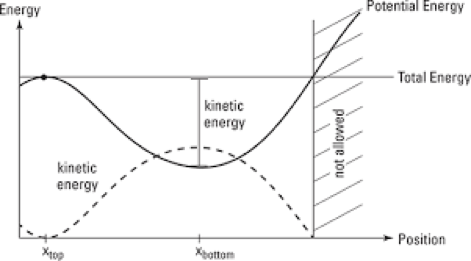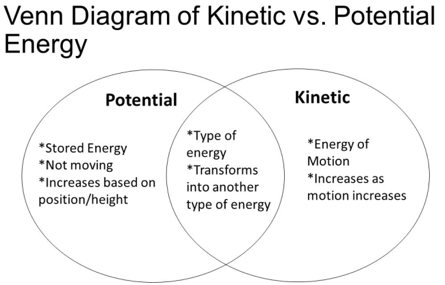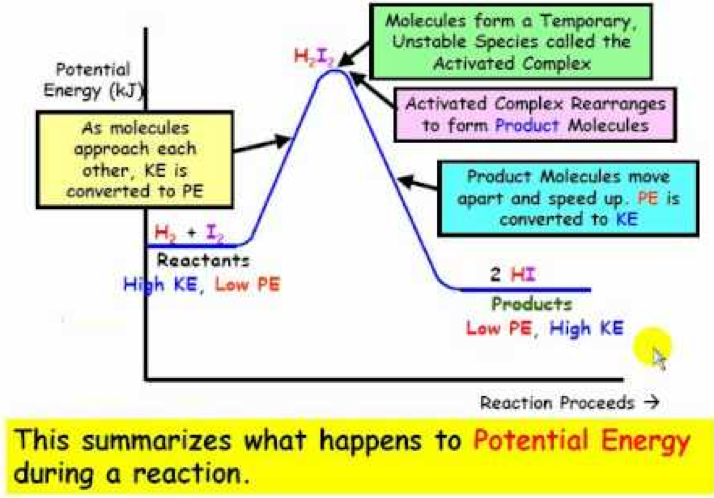# Kinetic Energy Diagram

When an object is moving, it possess an energy and such energy is called kinetic energy. To know more about kinetic energy, click here. Kinetic energy is explained along with its diagram here.

# Kinetic Energy Diagram### Kinetic energy Definition

• Kinetic energy is one of many types of energy that exist. This is energy generated because something is moving — the faster it’s going, the more kinetic energy it has. A person sitting has no kinetic energy, but a person running like a maniac has tremendous kinetic energy:

• The energy possessed by a body because of its motion, equal to one half the mass of the body times the square of its speed.

• The amount of kinetic energy KE of an object in translational motion is equal to one-half the product of its mass m and the square of its velocity v.

• Kinetic energy is given as follows,

Kinetic energy = ½(mv^2)